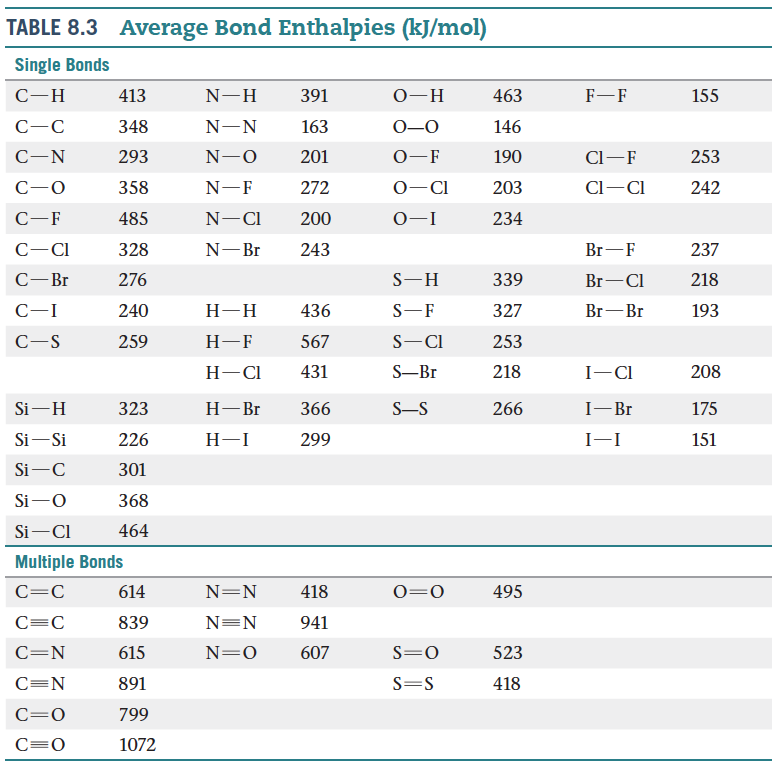# Problem: An important reaction for the conversion of natural gas to other useful hydrocarbons is the conversion of methane to ethane: 2 CH4(g) → C2H6(g) + H2(g)In practice, this reaction is carried out in the presence of oxygen, which converts the hydrogen produced to water: 2 CH4(g) + O2(g) → C2H6(g) + H2O(g)Use bond enthalpies to estimate ΔH for the first reaction.

###### FREE Expert Solution
93% (386 ratings)
###### Problem Details

An important reaction for the conversion of natural gas to other useful hydrocarbons is the conversion of methane to ethane: 2 CH4(g) → C2H6(g) + H2(g)

In practice, this reaction is carried out in the presence of oxygen, which converts the hydrogen produced to water: 2 CH4(g) + O2(g) → C2H6(g) + H2O(g)

Use bond enthalpies to estimate ΔH for the first reaction.Frequently Asked Questions

What scientific concept do you need to know in order to solve this problem?

Our tutors have indicated that to solve this problem you will need to apply the Bond Energy concept. You can view video lessons to learn Bond Energy. Or if you need more Bond Energy practice, you can also practice Bond Energy practice problems.